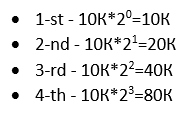Instance is a running strategy enabled for an instrument/timeframe. The Running Strategies tab indicates the complete list of instances.

Max Open Deals: Maximum number of open deals the strategy can open in one running instance.

Multiplicator: The following formula applied to calculate next deal volume:Multiplictor is applied to Buy and Sell open deals separately.

Example: Initial Order Volume = 10K, Multiplicator = 2If the initial order volume is changed then next deal volume is calculated based on the new order volume, there is no volume recalculation for deals opened before.

Both strategy parameters: Max Open Deals and Multiplicator mostly used in combination for so-called Martingale strategies.